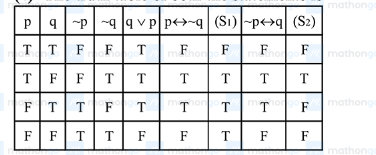# Given the following two statements :Question:

Given the following two statements :

$\left(S_{1}\right):(q \vee p) \rightarrow(p \leftrightarrow \sim q)$ is a tautology.

$\left(S_{2}\right): \sim q \wedge(\sim p \leftrightarrow q)$ is a fallacy. Then :

1. (1) both $\left(S_{1}\right)$ and $\left(S_{2}\right)$ are correct

2. (2) only $\left(S_{1}\right)$ is correct

3. (3) only $\left(S_{2}\right)$ is correct

4. (4) both $\left(S_{1}\right)$ and $\left(S_{2}\right)$ are not correct

Correct Option: , 4

Solution:

The truth table of both the statements is$\therefore \mathrm{S}_{1}$ is not tautology and

$\mathrm{S}_{2}$ is not fallacy.

Hence, both the statements $\left(S_{1}\right)$ and $\left(S_{2}\right)$ are not correct.